2019年08月31日

## 简介

《深入浅出Pytorch》理论上，根据万能近似定理（Universal Approximation Theorem）3，对于多层感知机来说，只要参数和隐含层的数量足够，就能够拟合任意的连续函数。此时，多层感知机是模型，模型固定了，就得调整参数 来使模型能够尽量拟合真实世界，调参问题 ==> 求损失函数最小值/梯度下降 ==> 复杂函数对参数微分 ==> 自动微分 ==> 正向传播+反向传播。

## 线性回归

1. 对于二维空间，分类器就是一条线，直线or 曲线
2. 对于三维空间，分类器就是一个面，平面or 曲面
3. 对于高维空间，xx

### 正向传播计算loss

【机器学习】代价函数（cost function）给出一个输入数据，我们的算法会通过一系列的过程得到一个估计的函数，这个函数有能力对没有见过的新数据给出一个新的估计，也被称为构建一个模型。假设有训练样本(x, y)，数据有两个特征x1,x2，模型为h，参数为θ，$\theta=(w1,w2,b)$，估计函数$h(x)=g(θ^T x)=g(w_1x_1+w_2x_2+b)$。即对x1,x2 施加一次线性变换 + 非线性变换，（$θ^T$表示θ的转置）。因为x1,x2 都是已知的，所以h(θ) 是一个关于θ的函数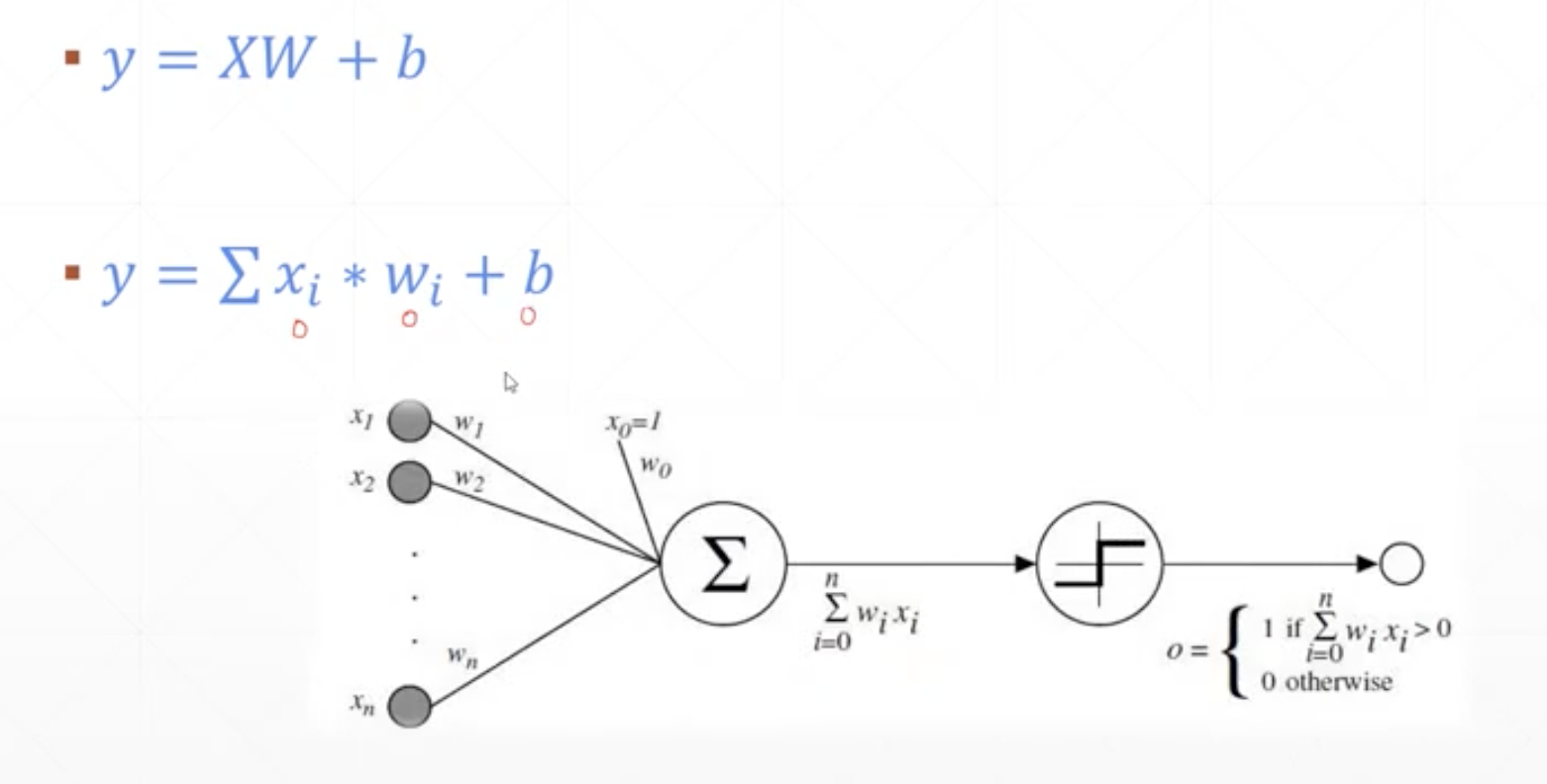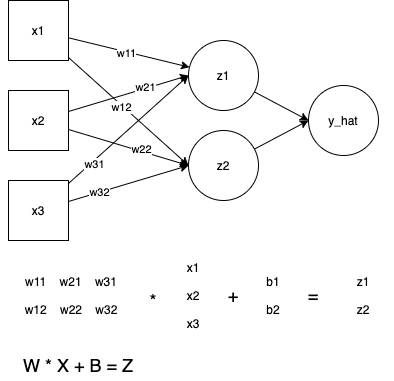1. 概况来讲，任何能够衡量模型预测出来的值h(θ)与真实值y之间的差异的函数都可以叫做代价函数C(θ)，如果有多个样本，则可以将所有代价函数的取值求均值，记做J(θ)。因此很容易就可以得出以下关于代价函数的性质：

• 对于每种算法来说，代价函数不是唯一的；
• 代价函数是参数θ的函数；
• 总的代价函数J(θ)可以用来评价模型的好坏，代价函数越小说明模型和参数越符合训练样本(x, y)；
• J(θ)是一个标量；
2. 当我们确定了模型h，后面做的所有事情就是训练模型的参数θ。那么什么时候模型的训练才能结束呢？这时候也涉及到代价函数，由于代价函数是用来衡量模型好坏的，我们的目标当然是得到最好的模型（也就是最符合训练样本(x, y)的模型）。因此训练参数的过程就是不断改变θ，从而得到更小的J(θ)的过程。理想情况下，当我们取到代价函数J的最小值时，就得到了最优的参数θ.例如，J(θ) = 0，表示我们的模型完美的拟合了观察的数据，没有任何误差。
3. 代价函数衡量的是模型预测值h(θ) 与标准答案y之间的差异，所以总的代价函数J是h(θ)和y的函数，即J=f(h(θ), y)。又因为y都是训练样本中给定的，h(θ)由θ决定，所以，最终还是模型参数θ的改变导致了J的改变。
4. 在优化参数θ的过程中，最常用的方法是梯度下降，这里的梯度就是代价函数J(θ)对θ1, θ2, ..., θn的偏导数。由于需要求偏导，我们可以得到另一个关于代价函数的性质：选择代价函数时，最好挑选对参数θ可微的函数（全微分存在，偏导数一定存在）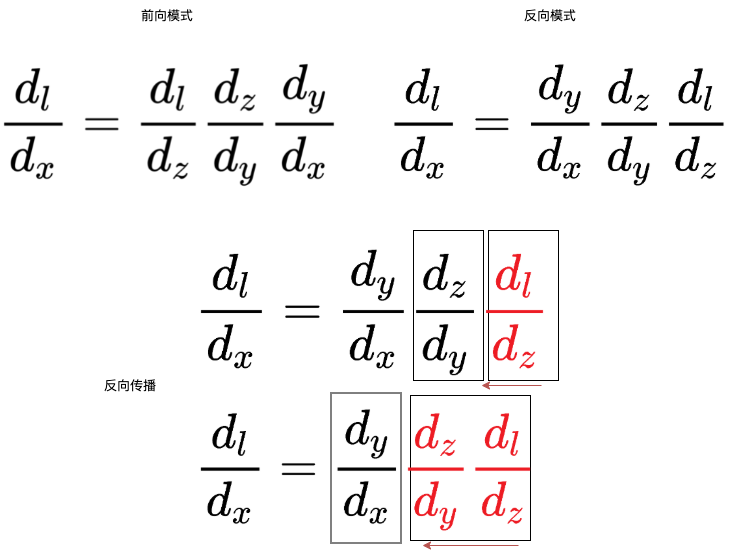y1 = x * w1 + b1
y2 = y1 * w2 + b2


dy2_dw1 可以用公式 推导直接算出来， 也可以 根据 $\frac{dy2}{dw1} = \frac{dy2}{dy1} * \frac{dy1}{dw1}$ 先计算 $\frac{dy2}{dy1}$ ，再使用 $\frac{dy2}{dy1} * \frac{dy1}{dw1}$ 计算 $\frac{dy2}{dw1}$。$\frac{dy2}{dw1}$ 偏导数的计算 先用到了 $\frac{dy2}{dy1}$，此为“反向”。y1 将 y2 对自己的梯度/导数 $\frac{dy2}{dy1}$ 和自己 对w1 梯度 $\frac{dy1}{dw1}$ 传给 w1，w1 将这两者 相乘 即可得到 y2（output） 对自己的梯度， 传播的是 output 对 y1 中间值的 偏导数，此为“反向传播”。PS： 对应到 《用python实现深度学习框架》，从计算图中作为结果的节点开始，依次从后向前，每个节点都将结果 对自己的雅克比矩阵 和 自己对父节点的雅克比矩阵 传给父节点，根据链式法则，父节点将这二者想乘就得到 结果对自己的雅克比矩阵。

### 优化算法：梯度下降法 ==> 求使得J极小的(w1,w2,b)

1. 从简单的地方开始。假设要最大化单变量函数。导数即某点切线的斜率，斜率为正，直线向上走；斜率为负，直线向下走。绝对值越大，直线越陡。如果想要到达山顶，应该沿切线上升的方向前进。如果切线的斜率较大，可以大步迈进；如果斜率接近零，应该小步小步往上爬，以免越过峰值。
2. 两个变量的函数，图像是一个曲面，很难定义切线的概念，用到了切平面，每个偏导数表示切平面上的一个方向。最陡的方向根据梯度确定，梯度为两个偏导数构成的向量。 所谓的梯度下降，如果要求函数最小值，就要沿负梯度的方向迈出一步，也就是下降最陡的方向。PS：梯度决定方向，学习率决定走多远，迭代式走到最小值（梯度为0）。

1. 使用目标函数的一阶导数，如梯度下降法。新解 = 老解 + 学习率 * 梯度
2. 使用目标函数的二阶导数，如牛顿法

 找一个 $\eta > 0$，使得 $\eta f’(x)$ 足够小，那么可以 用 $-\eta f’(x)$ 替换$\xi$ 代入 $f(x+\xi)\approx f(x)+\xi f’(x)$，得到
$f(x-\eta f'(x)) \approx f(x) - \eta f'(x)^2$

1. 首先对(w1,w2,b)赋值，这个值可以是随机的，也可以让(w1,w2,b)是一个全零的向量
2. 改变(w1,w2,b)的值，使得J(w1,w2,b)按梯度下降的方向进行减少，梯度方向由J(w1,w2,b)对(w1,w2,b)的偏导数确定。PS：求梯度 就是求损失值对每个参数的偏导数
3. $w1=w1+\sigma d_{w1}$ 依次得到 w1,w2,b 的新值，$\sigma$为学习率。 在代码上经常表示为 $w1=w1+\sigma * grad(w1)$

AI初识：为了围剿SGD大家这些年想过的那十几招

### 细节问题

#### 正向传播为什么需要非线性激活函数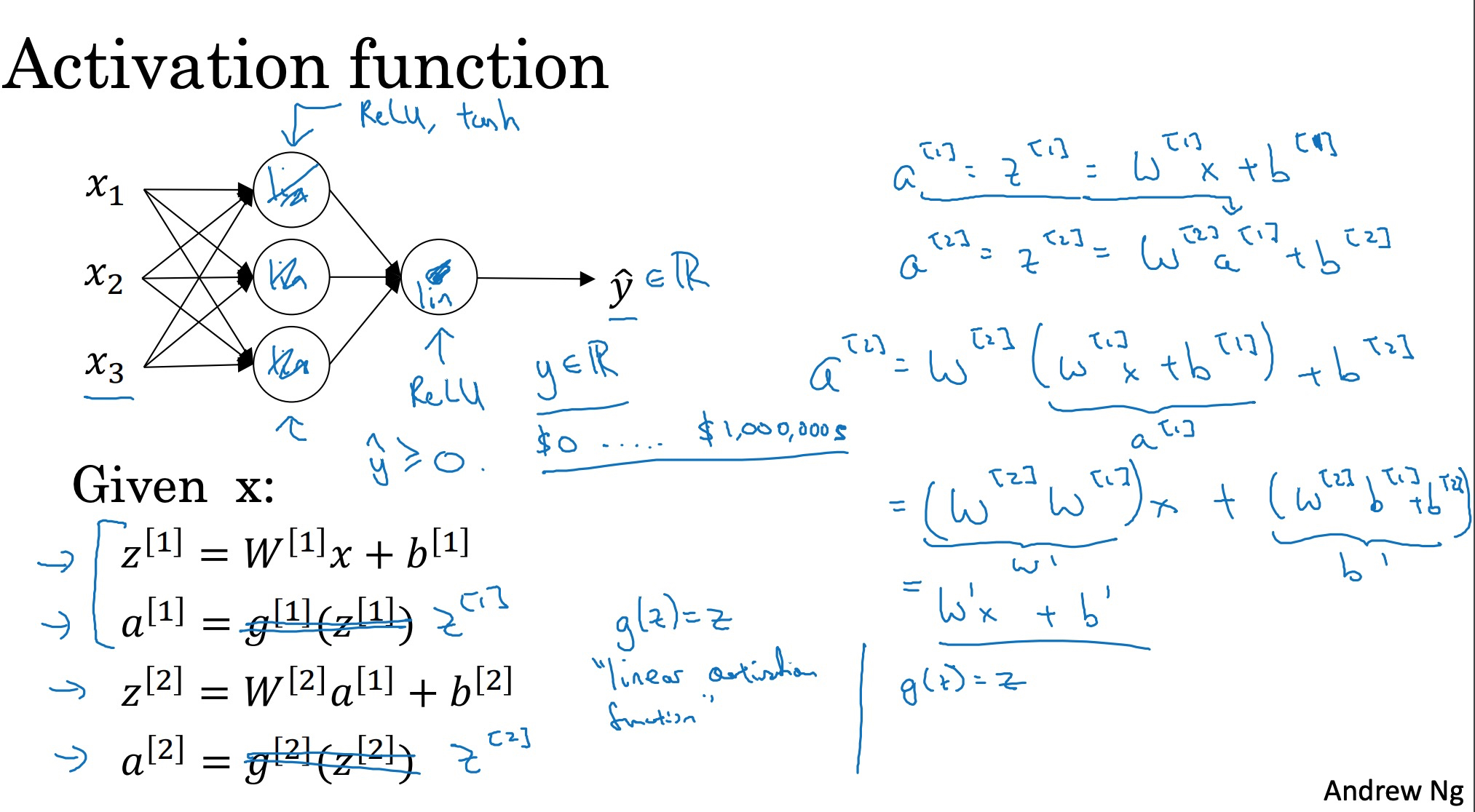sigmoid的作用是把输入信号从（-∞，+∞）的定义域映射到（0，1）的值域，且有处处可导的优良数学形式，方便之后的梯度下降学习过程，所以它成为了经常使用的激活函数。

#### 损失函数如何确定

1. log对数损失函数，PS：概率意义上，假设样本符合伯努利分布

$L(Y,P(Y|X))=-logP(Y|X)$
2. 平方损失函数 ，PS：概率意义上，假设误差符合高斯分布

$L(Y,h_\theta(X))=(Y-h\_\theta(x))^2$ $MSE(\theta)=MSE(X,h_\theta)=\frac{1}{m}\sum\_{i=1}^m(\theta^T.x^i-y^i)^2$
3. 指数损失函数
4. Hinge损失函数
5. 0-1损失函数
6. 绝对值损失函数

$L(Y,h_\theta(X))=|Y-h\_\theta(x)|$

https://zhuanlan.zhihu.com/p/46928319

## 从自动微分法来理解线性回归

《深入理解神经网络》用训练样本做输入向量，逐层计算直到计算出神经网络的输出，此过程称为前向传播。用网络输出和训练标签计算损失值。在训练样本和标签给定的情况下， 损失值可以视作<W,b> 的函数。用梯度下降法来更新<W,b> 以降低损失值，这就是神经网络的训练。 梯度下降法需要计算损失值 对 <W,b> 梯度，也就是损失值对 <W,b> 梯度，也就是损失值对 的偏导数。

1. 手动求解法(Manual Differentiation)，直接算出来 $\frac{df}{dx}=2xy$，用代码实现这个公式。然而大部分深度学习模型不好算公式。 PS: 一些课程 用实际的公式计算单层/两层 感知机 W的梯度
2. 数值微分法(Numerical Differentiation)，直接对原函数代入数值近似求解。直接 $\lim_{x \to x_0}\frac{h(x)-h(x_0)}{x-x_0}$。

3. 符号微分法(Symbolic Differentiation)，代替第一种手动求解法的过程，强调直接对代数进行求解，最后才代入问题数值；
4. 自动微分法(Automatic Differentiation)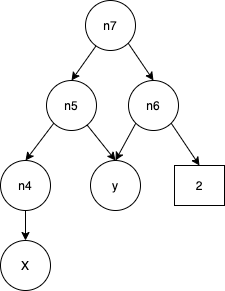[矩阵求导]神经网络反向传播梯度计算数学原理关于反向传播的数学原理，可能就不是那么好理解了，因为这里面需要用到矩阵的高级算法，一般的理工科数学的《线性代数》甚至《高等代数》里面都没有提到相关的内容，对于矩阵的微分是一个“三不管”的地带，但是这个内容又是深度学习神经网络中用得最多的数学原理，所以基本上已经超过了大多数高校学生的知识范围了。在这个时候，就要祭出张贤达的《矩阵分析》了。像数学工具这种内容，建议大家还是去看书，因为书作为几十年的经典教材，其推导过程，内容的完整性，认证的严密性都是经得起推敲的。网络文章只能帮大家启蒙一下，学几个术语，但是具体想深入了解细节，建议还是看书。

## 逻辑回归

$$f(x)=\theta^Tx=w_1x_1+w_2x_2+b$$ $$g(z)=\frac{1}{1+e^{-z}}$$

## 细节问题

### 激活函数为什么使用Sigmoid

1. 它的输入范围是正负无穷，而值刚好为（0，1），正好满足概率分布为（0，1）的要求。我们用概率去描述分类器，自然比单纯的某个阈值要方便很多
2. 它是一个单调上升的函数，具有良好的连续性，不存在不连续点

### 损失函数为什么使用对数损失函数

$h_\theta(x)$与y 只有0和1两个取值

#### 对数损失函数的直接意义

logistic回归详解(二）：损失函数（cost function）详解

$cost(h_\theta(x),y)= \begin{cases} -logh_\theta(x) & \text{if y=1} \\ -log(1-h_\theta(x)) & \text{if y=0} \end{cases}$

1. 如果此时$h_θ(x)=1$，$logh_θ(x)=0$，则单对这个样本而言的cost=0，表示这个样本的预测完全准确。
2. 如果此时预测的概率$h_θ(x)=0$，$logh_θ(x)=-\infty$，$-logh_θ(x)=\infty$，相当于对cost加一个很大的惩罚项。

$h_\theta(x)$ y cost
0 0 0
0 1 $\infty$
1 0 $\infty$
1 1 0

$cost(h_\theta(x),y)= -y\_ilogh\_\theta(x)- (1-y\_i)log(1-h\_\theta(x))$

$cost(h_\theta(x),y)= \sum\_{i=1}^m -y\_ilogh\_\theta(x)- (1-y\_i)log(1-h\_\theta(x))$

#### 对数损失函数的概率意义

$P(X=n)= \begin{cases} 1-p & \text{n=0} \\ p & \text{n=1} \end{cases}$

$f(x)=p^x(1-p)^{1-x}$

$P(data|p)= \prod_{i=1}^Nf(x\_i)= \prod\_{i=1}^Np^{x\_i}(1-p)^{1-x\_i}$

$lnP(data|p)= \sum\_{i=1}^Nx\_ilnp+(1-x\_i)(1-p)$

## 参数和超参数

parameters: $W^{},b^{},W^{},b^{},…$

1. learning rate
2. 梯度下降算法循环的数量
3. 隐层数
4. 每个隐层的单元数
5. 激活函数

### 为什么批量是一个超参数

1. 增大batch size能增大速度，但是很有限（主要是并行计算的优化）
2. 增大batch size能减缓梯度震荡，需要更少的迭代优化次数，收敛的更快，但是每次迭代耗时更长。
3. 增大batch size使得一个epoch所能进行的优化次数变少，收敛可能变慢，从而需要更多时间才能收敛（比如batch_size 变成全部样本数目）。

## 小结

1. 正向传播，线性 + 非线性函数（激活函数） 得到一个估计值
2. 反向传播，定义损失函数，通过（链式）求导 更新权重

1. 激活函数的选择
2. 损失函数的选择
3. 对损失函数及$\theta$链式求偏导数，涉及到矩阵的导数，根据偏导 + 学习率 调整$\theta$
4. 向量化上述过程
5. 使用python 中线程的 矩阵/向量计算的库 将上述过程代码化， 涉及到numpy的学习